Nonequispaced fast Fourier transform
Home
NFFT#### News

Matlab and Octave interfaces are now available as binaries for Windows and Linux on our download page.

Fast Fourier transforms (FFTs) belong to the '10 algorithms with the greatest influence on the development and practice of science and engineering in the 20th century'. The classic algorithm computes the discrete Fourier transform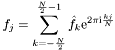for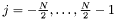and given complex coefficients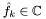. Using a divide and conquer approach, the number of floating point operations is reduced from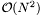for a straightforward computation to only. In conjunction with publicly available efficient implementations the fast Fourier transform has become of great importance in scientific computing.

However, two shortcomings of traditional schemes are the need for equispaced sampling and the restriction to the system of complex exponential functions. The NFFT (nonequispaced fast Fourier transform or nonuniform fast Fourier transform, NUFFT) is a C subroutine library for computing the nonequispaced discrete Fourier transform (NDFT) and its generalisations in one or more dimensions, of arbitrary input size, and of complex data.

More precisely,we collect the possible frequencies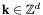in the multi-index set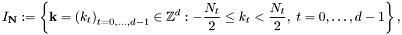where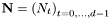is the multibandlimit, i.e.,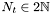. For a finite number of given Fourier coefficients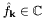,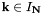, we consider the fast evaluation of the trigonometric polynomial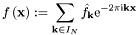at given nonequispaced nodes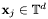,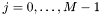, from the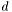-dimensional torus as well as the adjoint problem, the fast evaluation of sums of the form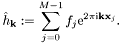In general, the adjoint NDFT is not the inverse transform of the NDFT.

The generalisations of the NFFT include

• NNFFT - nonequispaced in time and frequency fast Fourier transform,
• NFCT/NFST - nonequispaced fast (co)sine transform,
• NSFFT - nonequispaced sparse fast Fourier transform,
• FPT - fast polynomial transform,
• NFSFT - nonequispaced fast spherical Fourier transform,
• NFSOFT - nonequispaced fast Fourier transform on the rotation group

Furthermore, we consider the inversion of the above transforms by iterative methods.

The NFFT is a C subroutine library for computing the nonequispaced discrete Fourier transform (NDFT) in one or more dimensions, of arbitrary input size, and of complex data. New: A Matlab interface is part of the NFFT3. We believe that our library, which is free software, and based on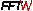(FFTW 3.x) should become the NFFT library of choice for most applications.

The NFFT package has been developed at the Mathematical Institute of the University of Lübeck, at the Mathematical Institute of the University Osnabrück and at the Faculty of Mathematics of the Chemnitz University of Technology by Jens Keiner, Stefan Kunis and Daniel Potts. Further contributions, in particular applications, are due to Dr. Markus Fenn, Steffen Klatt, Tobias Knopp and Antje Vollrath. The support for OpenMP was developed by Toni Volkmer. Many contributions to the release 3.3.* and later are done by Toni Volkmer and Michael Quellmalz.

If you have comments, questions, or suggestions regarding NFFT, don't hesitate to email at potts@mathematik.tu-chemnitz.de.counter